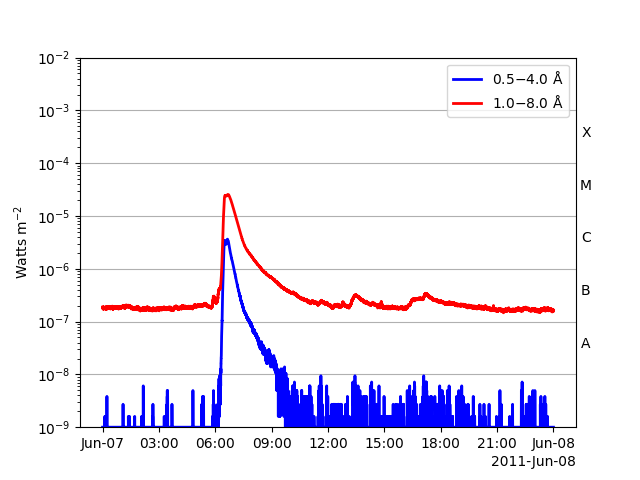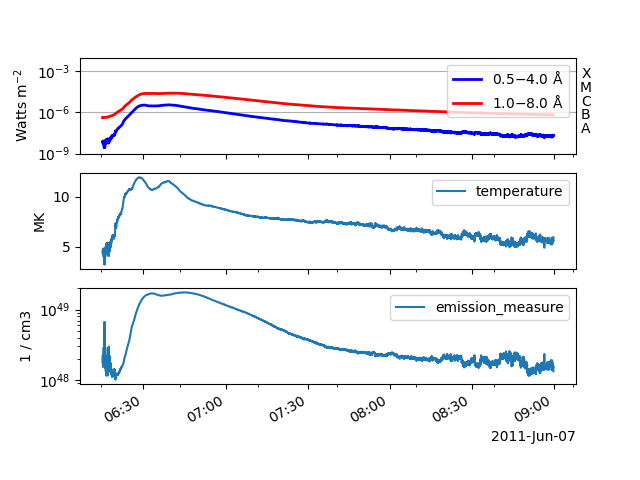# Calculate the GOES-XRS temperature and emission measure during a flare#

This example shows you how to estimate the GOES-XRS isothermal temperature and emission measure during a solar flare. This is done using the observed flux ratio of the short (0.5-4 angstrom) to long (1-8 angstrom) channels, based on the methods described in White et al. (2005). The functionality available here is the same as the functionality available in SSWIDL.

```import matplotlib.pyplot as plt

from sunpy import timeseries as ts
from sunpy.data.sample import GOES_XRS_TIMESERIES

from sunkit_instruments import goes_xrs
```

Let’s begin by creating a GOES-XRS timeseries. We can use the sample data here to load in an example of a flare.

```goes_ts = ts.TimeSeries(GOES_XRS_TIMESERIES)
goes_ts.plot()
``````<Axes: ylabel='Watts m\$^{-2}\$'>
```

The estimation is only valid for large fluxes (i.e. during a flare), so let’s truncate the timeseries over the time of the flare.

```goes_flare = goes_ts.truncate("2011-06-07 06:15", "2011-06-07 09:00")
```

Now let’s calculate the temperature and emission measure estimates from these channel fluxes. We can do this by using the function `sunkit_instruments.goes_xrs.calculate_temperature_emiss()` which takes a sunpy.timeseries.XRSTimeSeries and returns a new GenericTimeSeries which contains both the respective temperature and emission measure values.

```goes_temp_em = goes_xrs.calculate_temperature_em(goes_flare)
```

We can see that goes_temp_em is now a timeseries that contains the temperature and emission measure by printing out the column names.

```print(goes_temp_em.columns)
```
```['temperature', 'emission_measure']
```

Now let’s plot these all together.

```fig, (ax1, ax2, ax3) = plt.subplots(3, sharex=True)
goes_flare.plot(axes=ax1)
goes_temp_em.plot(columns=["temperature"], axes=ax2)
goes_temp_em.plot(columns=["emission_measure"], axes=ax3)
ax3.set_yscale("log")
plt.show()
```Total running time of the script: (0 minutes 4.107 seconds)

Gallery generated by Sphinx-Gallery